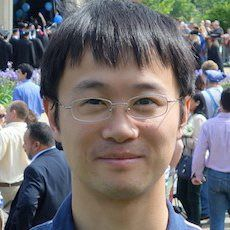# Events

## DMS Stochastic Analysis Seminar

Time: Nov 08, 2022 (02:30 PM)
Location: 356 Parker Hall

Details:Speaker: Jingyu Huang, University of Birmingham, UK

Title: Fourier transform method in stochastic differential equation (SPDE)

Abstract: We consider the Fourier transform method in stochastic heat equation on $$\mathbb{R}^d$$

$$\frac{\partial \theta}{\partial t} = \frac{1}{2} \Delta \theta(t,x) + \theta(t,x) \dot{W}(t,x).$$

We study the existence and uniqueness of the solution under Fourier mode.

Then we apply the similar approach to the turbulent transport of a passive scalar quantity in a stratified, 2-D random velocity field. It is described by the stochastic partial differential equation
$$\partial_t \theta(t,x,y) = \nu \Delta \theta(t,x,y) + \dot{V}(t,x) \partial_y \theta(t,x,y), \quad t\ge 0\:\: \text{and}\:\: x,y\in \mathbb{R},$$
where $$\dot{V}$$ is some Gaussian noise. We show via a priori bounds that, typically, the solution decays with time. The detailed analysis is based on a probabilistic representation of the solution, which is likely to have other applications as well. This is based on joint work with Davar Khoshnevisan from University of Utah.